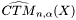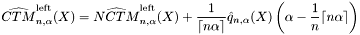#Boost C++ Libraries

...one of the most highly regarded and expertly designed C++ library projects in the world.

## Struct template coherent_tail_mean_impl

boost::accumulators::impl::coherent_tail_mean_impl — Estimation of the coherent tail mean based on order statistics (for both left and right tails).

## Synopsis

```// In header: <boost/accumulators/statistics/tail_mean.hpp>

template<typename Sample, typename LeftRight>
struct coherent_tail_mean_impl {
// types
typedef numeric::functional::average< Sample, std::size_t >::result_type float_type;
typedef float_type                                                       result_type;

// construct/copy/destruct
coherent_tail_mean_impl(dont_care);

// public member functions
template<typename Args> result_type result(Args const &) const;
};```

## Description

The coherent tail meanis equal to the non-coherent tail meanplus a correction term that ensures coherence in case of non-continuous distributions.

Equation 1.12.Equation 1.13.### `coherent_tail_mean_impl` public construct/copy/destruct

1. `coherent_tail_mean_impl(dont_care);`

### `coherent_tail_mean_impl` public member functions

1. `template<typename Args> result_type result(Args const & args) const;`